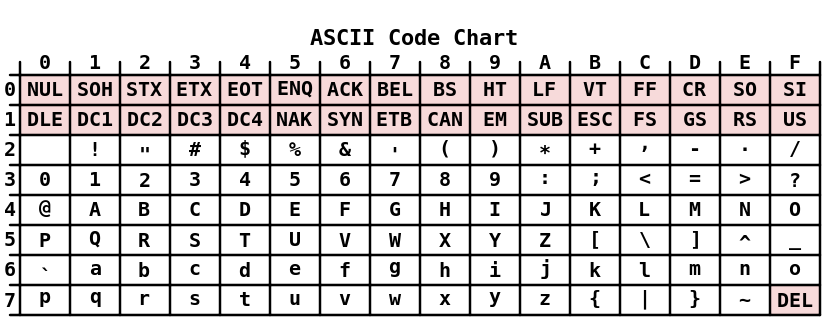# Manipulate Unicode in Go

We work with string every day. We work we character sets every day. But how much do we know about ASCII, UTF-8, Unicode?

There is the Caesar cipher method:

The method in which each letter in the plaintext is replaced by a letter some fixed number of positions down the alphabet. The method is named after Julius Caesar, who used it in his private correspondence.

Assume we have “hello worldz” and want encode it with shifting by 1.

String holds a sequence of runes. Rune is a Unicode “code point” in Go language and represented as an alias for the type int32.

If we want a shift by 1 just add 1 until … until we reach `z` and then we just add the difference to `a` minus 1:

 `````` 1 2 3 4 5 6 7 8 9 10 11 12 13 14 15 16 `````` ``````func main() { str := "hello worldz" rs := make([]rune, 0, len(str)) for _, r := range str { if r < 'a' { rs = append(rs, r) continue } enc := r + rune(1) if enc > 'z' { enc = 'a' + (enc - 'z' - 1) } rs = append(rs, enc) } fmt.Println(string(rs)) } ``````

ifmmp!xpsmea

We lost our space. We have to not encode symbols. `a` has decimal number 97 and space is 32 so we can skip everything that is below 97:

 `````` 1 2 3 4 5 6 7 8 9 10 11 `````` ``````for _, r := range str { if r < 'a' { rs = append(rs, r) continue } enc := r + rune(1) if enc > 'z' { enc = 'a' + (enc - 'z' - 1) } rs = append(rs, enc) } ``````

ifmmp xpsmea

But what if we have to encode “Hello Worldz{}" ? Then we have to care about a case.

Now we have to dig deepper. How can we describe all that symbols? English keyboard layout? Or probably the ASCII character set.

The ASCII character set defines 128 characters (0 to 127 decimal, 0 to 7F hexadecimal, and 0 to 177 octal).The first 32 characters are control characters, unprintable. Alphabet, upper case, `A-Z`: 65-90. Alphabet, lower case, `a-z`: 97-122. All others are symbol characters and digits.

We care only about alphabet sybmols, and skip shifting other. Howerer we should shift upper and lower case separate.

 ``````1 2 3 4 5 6 7 8 `````` `````` func isUpper(r rune) bool { return r >= 'A' && r<='Z' } func isLower(r rune) bool { return r >= 'a' && r<='z' } ``````

Entire pregram:

 `````` 1 2 3 4 5 6 7 8 9 10 11 12 13 14 15 16 17 18 19 20 21 22 23 24 25 26 27 28 29 30 31 32 33 34 35 `````` ``````func main() { str := "Hello Worldz{}" rs := make([]rune, 0, len(str)) for _, r := range str { rs = append(rs, encode(r, 1)) } fmt.Println(string(rs)) } func encode(r rune, shift int) rune { if isUpper(r) { return encodeLetter(r, shift, 'A', 'Z') } if isLower(r) { return encodeLetter(r, shift, 'a', 'z') } return r } func isUpper(r rune) bool { return r >= 'A' && r <= 'Z' } func isLower(r rune) bool { return r >= 'a' && r <= 'z' } func encodeLetter(r rune, shift int, lo, hi rune) rune { enc := r + rune(shift) if enc > hi { enc = lo + (enc - hi - 1) } return enc } ``````

Let’s change our task from Ceasar’s Cipher to replace letters with their alphabet number: `H -> 8`, `e -> 5`.

Hello Worldz{} -> 85121215 23151812426{}

That means we consider upper and lower case letter(`H`, `h`) as the same number(`8`). So we just work with every letter as a lower case letter just conver upper to lower. Then we calculate `letter - 'a' + 1` as letter number, then add it to `'0'`

But it a bit complicated with `l` cause the number is 12. So we represent it as `[]{'1','2'}`:

 `````` 1 2 3 4 5 6 7 8 9 10 11 12 `````` ``````func encodeLetter(r rune) []rune { rs := make([]rune, 0) number := int(r-'a') + 1 for (number / 10) > 0 { k := number / 10 rs = append(rs, '0'+rune(k)) number -= k * 10 } return append(rs, '0'+rune(number%10)) } ``````

Full program:

 `````` 1 2 3 4 5 6 7 8 9 10 11 12 13 14 15 16 17 18 19 20 21 22 23 24 25 26 27 28 29 30 31 32 33 34 35 36 37 38 39 40 41 42 43 44 `````` ``````func main() { str := "Hello Worldz{}" rs := make([]rune, 0, len(str)) for _, r := range str { rs = append(rs, encode(r)...) } fmt.Println(string(rs)) } func encode(r rune) []rune { if isUpper(r) { return encodeLetter(toLower(r)) } if isLower(r) { return encodeLetter(r) } return []rune{r} } func isUpper(r rune) bool { return r >= 'A' && r <= 'Z' } func isLower(r rune) bool { return r >= 'a' && r <= 'z' } func toLower(r rune) rune { return 'a' + r - 'A' } func encodeLetter(r rune) []rune { rs := make([]rune, 0) number := int(r-'a') + 1 for (number / 10) > 0 { k := number / 10 rs = append(rs, '0'+rune(k)) number -= k * 10 } return append(rs, '0'+rune(number%10)) } ``````

The Absolute Minimum Every Software Developer Absolutely, Positively Must Know About Unicode and Character Sets (No Excuses!)